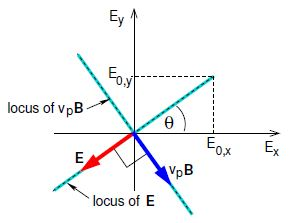Mathematics

# Explain Linear Polarisation

In linear polarisation the two phase constants are equal, by ϐy = ϐx ≡ ϐ, and so

Re {R (r, t) = (x E0,x + y E0,y) cos (kz – wt + ϐ)

The locus of the tip of the E vector performs simple harmonic motion along a line at angle θ = arctan (E0,y/E0,x) to the Ex-axis in the Ex – Ey plane. This means that the EM wave is linearly polarised (plane polarised) such that the electric field direction is at angle θ to the x-axis. Below Figure already showed E and B for a linearly polarised wave. Linearly polarised quasi-monochromatic plane waves may be produced by passing unpolarised light through a monochromatic filter and then through a polariser such as Polaroid film, or better still by passing a laser beam through a polariser.Figure: The locus of the tip of the E vector and the vp B vector in the Ex—Ey plane for the case of linear polarisation.

A single photon from spontaneous decay can initiate maser action. As it propagates through the region of the molecular cloud having the population inversion the photon triggers repeated stimulated emission of essentially identical photons in phase with itself, as do the photons produced by stimulated emission. There is thus a cascade or avalanche in which the number of photons build up exponentially with distance, and because the path-lengths through the cloud are huge the resulting brightness (intensity per unit solid angle) of coherent microwave radiation is sufficiently high to be observable with radio telescopes.